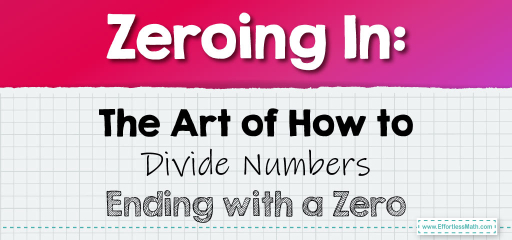# Zeroing In: The Art of How to Divide Numbers Ending with a Zero

Numbers ending in one or more zeros have unique division properties that can simplify calculations. Recognizing these properties can make division quicker and more intuitive. Let's explore the nuances of dividing numbers that end in a zero.

Key Insight:

When a number ends in a zero, it's a multiple of $$10$$. The more zeros it ends with, the higher the power of $$10$$ it's a multiple of. This property can be leveraged to simplify division.## Dividing Numbers Ending in a Zero

### Example 1: Dividing by a Single-digit Number

Divide $$500$$ by $$5$$.

Solution Process:

Instead of directly dividing, you can simplify. Divide $$50$$ by $$5$$ to get $$10$$, then account for the ending zero.

The result is $$100$$.

The Absolute Best Book for 5th Grade Students

### Example 2: Dividing by a Number Ending in Zero

Divide $$600$$ by $$20$$.

Solution Process:

Remove the common zero from both numbers, turning the problem into $$60 \div 2$$.

The result is $$30$$.

### Example 3: Dividing a Larger Number Ending in Zeros

Divide $$8,000$$ by $$40$$.

Solution Process:

Remove the common zeros, simplifying the problem to $$80 \div 4$$.

The result is $$20$$, and then account for the ending zero to get $$200$$.

Numbers ending in zeros have a structure that can be harnessed for easier division. By recognizing the multiples of $$10$$ and simplifying the division process, you can quickly and efficiently find the quotient. This approach not only speeds up calculations but also deepens understanding of the relationships between numbers. Embrace the power of zero, and watch your division skills soar!

### Practice Questions:

1. Divide $$700$$ by $$7$$.

2. What is $$4,000$$ divided by $$20$$?

3. Divide $$9,000$$ by $$30$$.

4. What is the result of $$5,000$$ divided by $$50$$?

5. Divide $$10,000$$ by $$200$$.

A Perfect Book for Grade 5 Math Word Problems!

1. $$100$$

2. $$200$$

3. $$300$$

4. $$100$$

5. $$50$$

The Best Math Books for Elementary Students

### What people say about "Zeroing In: The Art of How to Divide Numbers Ending with a Zero - Effortless Math: We Help Students Learn to LOVE Mathematics"?

No one replied yet.

X
51% OFF

Limited time only!

Save Over 51%

SAVE $15 It was$29.99 now it is \$14.99